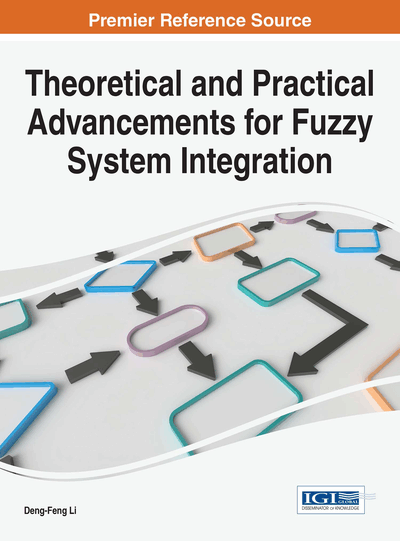# Intuitionistic Fuzzy Distance-Based Intuitionistic Fuzzy TOPSIS Method and Application to MADM

Jiangxia Nan (Guilin University of Electronic Technology, China), Ting Wang (Guilin University of Electronic Technology, China) and Jingjing An (Fuzhou University, China)
DOI: 10.4018/978-1-5225-1848-8.ch004

## Abstract

In this paper, an intuitionistic fuzzy (IF) distance measure between two triangular intuitionistic fuzzy numbers (TIFNs) is developed. The metric properties of the proposed IF distance measures are also studied. Then, based on the IF distance, an extended TOPSIS is developed to solve multi-attribute decision making (MADM) problems with the ratings of alternatives on attributes of TIFNs. In this methodology, the IF distances between each alternative and the TIFN positive ideal-solution are calculated as well as the TIFN negative ideal-solution. Then the relative closeness degrees obtained of each alternative to the TIFN positive ideal solution are TIFNs. Based on the ranking methods of TIFNs the alternatives are ranked. A numerical example is examined to the validity and practicability of the method proposed in this paper.
Chapter Preview
Top

## Introduction

In the application of fuzzy numbers, distance measures between fuzzy numbers play a very important role. Thus some researchers of Cheng (1998), Chakraborty (2006), Guha and Chakraborty (2010), Tran and Duckstein (2002), Voxman (1998), Xu and Li (2001) have focused their attention to compute the distances between fuzzy numbers (Cheng, 1998; Chakraborty, 2006; Guha & Chakraborty, 2010; Tran & Duckstein, 2002; Voxman, 1998; Xu & Li, 2001). Atanassov (1999) proposed the intuitionistic fuzzy (IF) sets, which is an extension of the fuzzy set and it is better than fuzzy set in dealing with vagueness and uncertainty of more flexible and practical. Based on the study of distance measures between fuzzy numbers, some researchers studied the distance between IF sets (Grzegorzewski, 2004; Szmidt & Kacprzyk, 2000; Wang & Xin, 2005). As a specialization of IF-set, the concept of intuitionistic fuzzy numbers (IFNs) can express information more abundant and flexible than the fuzzy number. However, there is very little investigation on distance measure between IFNs. Grzegorzewski (2003) studied the distance of IFNs, which is expressed as crisp values. It is unreasonable, the reason is that from a viewpoint of logic, a distance measure between IFNs should be an IFN. In other word, the uncertainty or hesitation or lack of knowledge presented in defining IFN should inherently be involved in their corresponding distance measures. Guha and Chakraborty (2010) studied the distance measure between two IFNs, which is IFN. In this paper, extending the fuzzy distance given by Tran and Duckstein (2002), a new IF distance measure between two triangular intuitionistic fuzzy numbers (TIFNs) defined by Li (2010) is proposed based on interval difference. Here the distance between two TIFNs is also TIFN.

## Complete Chapter List

Search this Book:
Reset